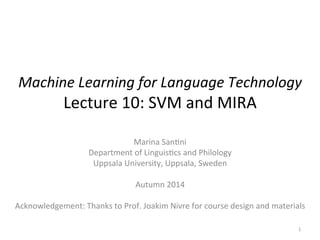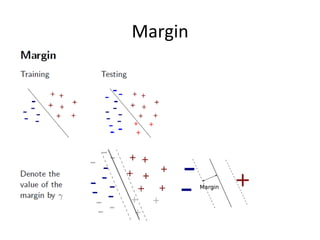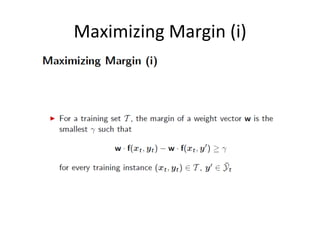Successfully reported this slideshow.

# Lecture 10: SVM and MIRA×

# Lecture 10: SVM and MIRA

Outline: margin, maximizing margin, the norm, support vectors machines, SVM, Margin Infused Relaxed Algorithm, MIRA

Outline: margin, maximizing margin, the norm, support vectors machines, SVM, Margin Infused Relaxed Algorithm, MIRA

### Lecture 10: SVM and MIRA

1. 1. Machine Learning for Language Technology Lecture 10: SVM and MIRA Marina San5ni Department of Linguis5cs and Philology Uppsala University, Uppsala, Sweden Autumn 2014 Acknowledgement: Thanks to Prof. Joakim Nivre for course design and materials 1
2. 2. Margin
3. 3. Maximizing Margin (i)
4. 4. Maximizing Margin (ii)
5. 5. Maximizing Margin (iii)
6. 6. Max Margin = Min Norm
7. 7. Maximizing the margin Linear Classifiers: Repe55on & Extension 7 • The no5on of margin: a way of predic5ng what it will be a good separa5on on the test set. • Intui5vely, if we make the margin between opposite groups as wide as possible, our chances to guess correct in the test set should increase. • the generaliza5on error on unseen test data is propor5onal to the inverse of the margin: the larger the margin, the smaller the generaliza5on error
8. 8. Support Vector Machines (SVM) (i)
9. 9. Support Vector Machines (SVM) (ii)
10. 10. Margin Infused Relaxed Algorithm (MIRA)
11. 11. MIRA
12. 12. Perceptron vs. SVMs/MIRA Linear Classifiers: Repe55on & Extension 12 Perceptron SVMs/MIRA If the training set is separable by some margin, the Perceptron will find a weight vector that separates the data, but it will not necessarily pick up the vector that maximizes the margin. If we are lucky, it will be a vector with the largest margin, but there will be no guarantee. SVMs/MIRA want a weight vector that maximizes the margin to 1. Here the margin is normalized to 1. So we put a constraint on the weight vector saying that the weight should be such that when you computes the norm we should get 1. We keep the margin fixed and minimize the norm. That is, we want the smallest weight vector that gives us margin 1. We do not minimize the norm, we minimize the norm squared divided by 2 to make the math easier (trust the people who suggested this J )
13. 13. Summary
14. 14. The end